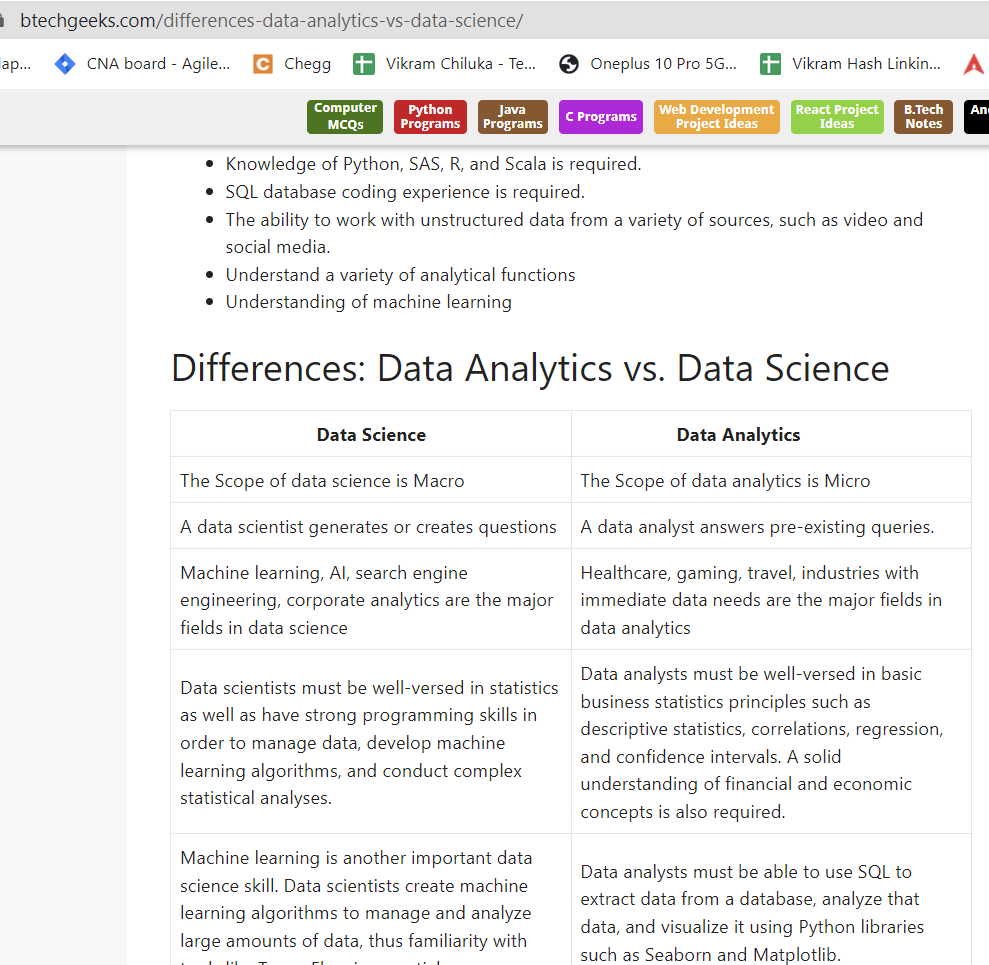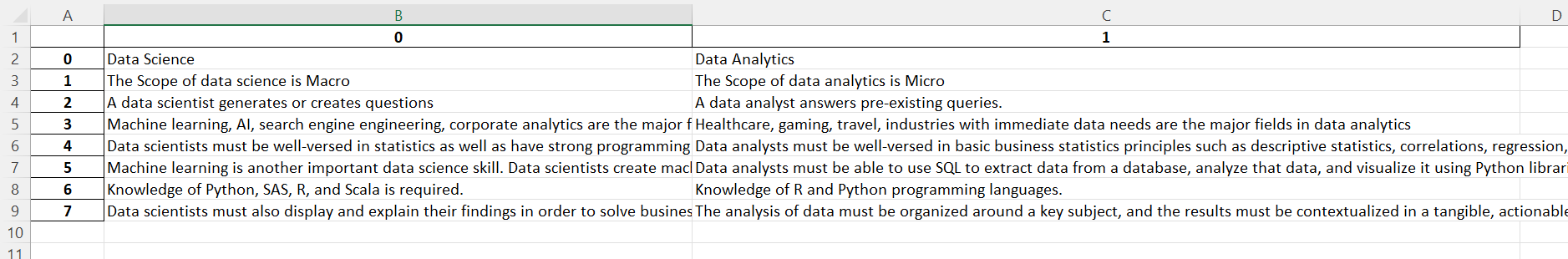# Python Program to Convert an HTML Table into excel

HTML Table To Excel: For managing massive amounts of tabular data, MS Excel is a powerful resource. It can be especially helpful for data visualization, analysis, and complex calculations. In this tutorial, we will look at how to extract a table from a webpage and save information in Excel format.

Table manipulation can be done using the Python package Pandas.  The table from the web page needs to be saved in a Pandas data frame as our initial action.  The read_html() method returns a list of data frames, each of which represents a web page table. This article has a detailed explanation of the steps involved in converting HTML tables to Excel using Python. Scroll down to find more.

Prerequisites:

## What is HTML Table?

A Web page’s HTML structure for constructing rows and columns. The Table tag specifies the overall table, whereas the Table Row (TR) tag specifies how each row is constructed. The real data is defined by the Table Data (TD) tag. Tables were commonly utilized for almost every element on the page prior to HTML5.

Given a website that contains HTML table, the task is to convert it to excel using python pandas## Program to Convert an HTML Table into excel in Python

Below are the ways to convert an HTML Table to excel in Python using Pandas:

### Method #1: Converting HTML table to Pandas data frame

The pandas read_html() function converts an HTML table into a pandas DataFrame in a rapid and easy manner. This tool can be useful for quickly combining tables from different websites without having to figure out how to scrape the site’s HTML. However, there may be some difficulties in cleaning and structuring the data prior to analysis.

Approach:

• Import the pandas module using the import keyword
• Give the webpage URL link and store it in a variable
• Create a Pandas dataframe using the table data.
• Print the table data using the print statement.
• The Exit of the Program.

Below is the Implementation:

```# import the pandas module using the import keyword
import pandas as pd

#give the webpage url link and store it in a variable
webpage_url = "https://btechgeeks.com/differences-data-analytics-vs-data-science/"

# Create a Pandas dataframe using the table data.

# print the table data using the print statement
print(table_data)
```

Output:

index 0 1
0 Data Science Data Analytics
1 The Scope of data science is Macro The Scope of data analytics is Micro
2 A data scientist generates or creates questions A data analyst answers pre-existing queries.
3 Machine learning, AI, search engine engineering, corporate analytics are the major fields in data science Healthcare, gaming, travel, industries with immediate data needs are the major fields in data analytics
4 Data scientists must be well-versed in statistics as well as have strong programming skills in order to manage data, develop machine learning algorithms, and conduct complex statistical analyses. Data analysts must be well-versed in basic business statistics principles such as descriptive statistics, correlations, regression, and confidence intervals. A solid understanding of financial and economic concepts is also required.
5 Machine learning is another important data science skill. Data scientists create machine learning algorithms to manage and analyze large amounts of data, thus familiarity with tools like TensorFlow is essential. Data analysts must be able to use SQL to extract data from a database, analyze that data, and visualize it using Python libraries such as Seaborn and Matplotlib.
6 Knowledge of Python, SAS, R, and Scala is required. Knowledge of R and Python programming languages.
7 Data scientists must also display and explain their findings in order to solve business challenges. As a result, data science sits at the intersections of computer science, mathematics, statistics, and business intelligence. The analysis of data must be organized around a key subject, and the results must be contextualized in a tangible, actionable fashion that provides commercial value. Because data analysts frequently collaborate with business stakeholders, they may even assist in the creation of client pitches and business performance dashboards. Data analysts in these situations must be able to visualize data using tools such as Microsoft Power BI or Tableau.

### Method #2: Converting the HTML Web Page to Excel

to_excel() function:

To export the DataFrame to an excel file, use the to excel() method. The target file name must be specified when writing a single object to an excel file. If we wish to write to many sheets, we must first construct an ExcelWriter object with the target filename and then indicate the sheet in the file we want to write to. The unique sheet name can also be used to write numerous sheets. All modifications made to the data written to the file must be saved.

We utilize Pandas’ to_excel() function for this, passing the filename as an argument.

Approach:

• Import the pandas module using the import keyword
• Give the webpage URL link and store it in a variable
• Create a Pandas dataframe using the table data.
• Store the above-created dataframe as an excel file using the to_excel() function
• The Exit of the Program.

Below is the Implementation:

```# import the pandas module using the import keyword
import pandas as pd

#give the webpage url link and store it in a variable
webpage_url = "https://btechgeeks.com/differences-data-analytics-vs-data-science/"

# Create a Pandas dataframe using the table data.